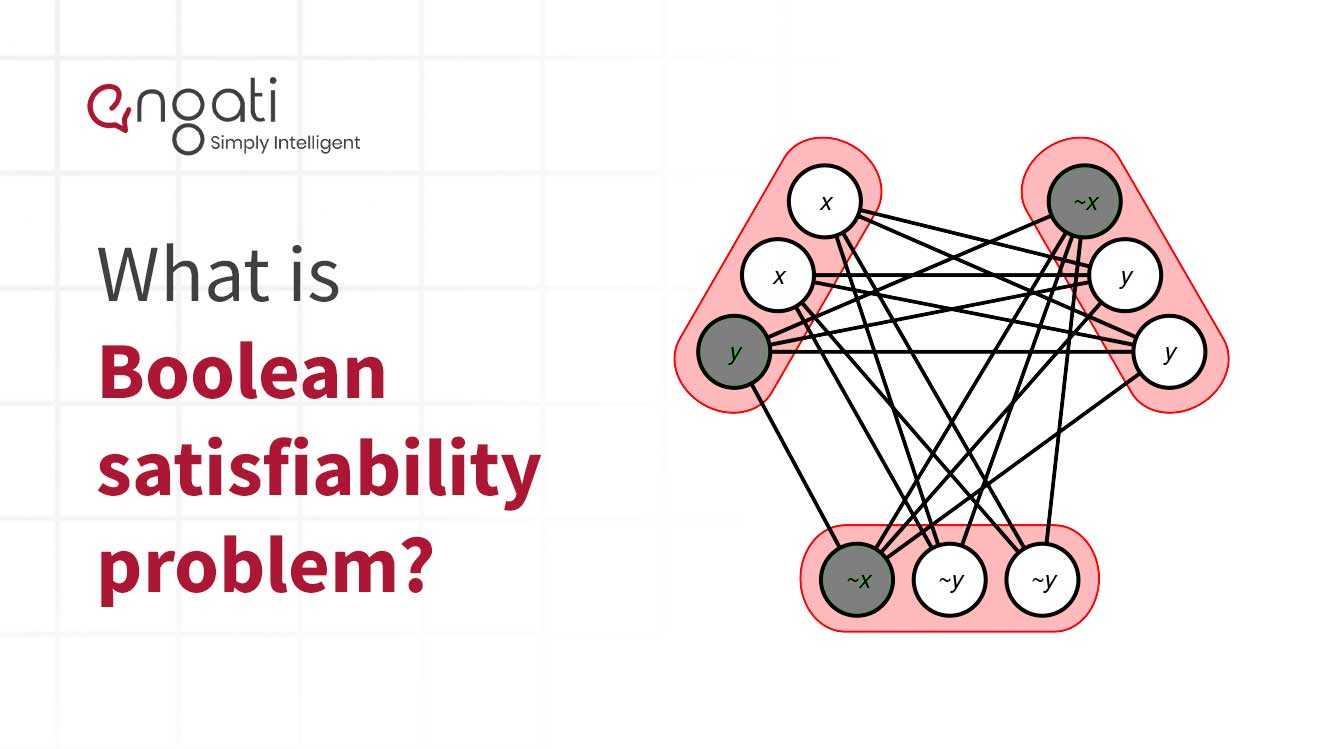# Boolean satisfiability problem## What is boolean satisfiability problem?

The Boolean satisfiability problem (B-SAT) is a problem solver containing binary variables connected by logical relations such as OR and AND using SAT formulas.

In other words, it asks whether the variables of a given Boolean formula can be consistently replaced by the values TRUE or FALSE in such a way that the formula evaluates to TRUE. If this is the case, the formula is called satisfiable. On the other hand, if no such assignment exists, the function expressed by the formula is FALSE for all possible variable assignments and the formula is unsatisfiable.

## What are some of boolean satisfiability problem’s initial operators?

In order to ensure that we are able to satisfy any formula first we need to have the necessary operators to represent it. For that purpose, we can use 0 and 1 values to represent False and True values respectively. As it is explained by George Boole in 1847, we can use three intuitive operators where:

• x and y = min(x, y)
• x or y = max(x, y)
• not x = 1- x

To make things easier, we’ll use a new operator to replace the or and not; Choice, and when you type (x | y | z) = 1 means you can choose which x, y or z is 1, but only one and exactly one. In fact, you could type (x | y) = 1, which means x = 1 - y = not y.

Considering the · operator A·B = A and B, now we are prepared to show beautiful formulas…

And…, [(x1 | … | xn) = 1] ↔ [x1 + … + xn = 1]. Later, we will use another notation, but now let’s study what can we do with this operator.

Proposition. Having the next equation (A|B|C)·(B|D|E)·(C|E|F)=1, where A, B, …, F are Boolean variables, the following statements are fulfilled:

B = not A and not D

F = A ↔ D

So we can construct the next theorem: for every x, y Boolean values:

(not x | x and y | z1)·(x and y | not y | z2)·(z1 | z2 | x ↔ y) = 1

So, as corollary: we can represent every Boolean formula as a conjunction (·) of choices of Boolean variables.

You can consider some other studies about how different problems (interesting for the Computer Science) could be represented.Request a Demo!
Get started on Engati with the help of a personalised demo.
This is some text inside of a div block.
This is some text inside of a div block.
This is some text inside of a div block.
This is some text inside of a div block.
*only for sharing demo link on WhatsApp
Thanks for the information.
We will be shortly getting in touch with you.
Oops! something went wrong!
For any query reach out to us on contact@engati.comI am looking for a conversational AI engagement solution for the web and other channels.I would like for a conversational AI engagement solution for WhatsApp as the primary channelI am an e-commerce store with Shopify. I am looking for a conversational AI engagement solution for my businessI am looking to partner with Engati to build conversational AI solutions for other businessesYou're a step away from building your Al chatbot

How many customers do you expect to engage in a month?

Less Than 2000

2000-5000

More than 5000Thanks for the information.

We will be shortly getting in touch with you.